Home > CCA2 > Chapter 9 > Lesson 9.3.3 > Problem9-105

9-105.
1. Due to natural variability in manufacturing, a 12-ounce can of soda does not usually hold exactly 12 ounces of soda. The quality control department at a soda factory allows cans to hold a little more or a little less. According to specifications, a soda-can filling machine must fill each can with a mean of 12 ounces of soda and a standard deviation of 0.33 ounces. The amount of soda in a can filled by filling machines can often be modeled with a normal distribution. Homework Help ✎

1. Use your calculator to create a graph of a normal distribution using normalpdf. Sketch the graph. An appropriate value for the maximum of the relative frequency axis is Ymax = 1.5.

2. How often do you actually get a 12 oz can of soda containing more than 12 oz?

3. What percent of cans contain between 11.5 and 12.5 ounces of soda? Shade your diagram from part (a) to represent these cans.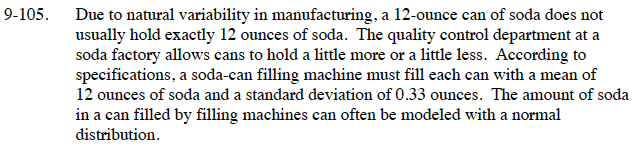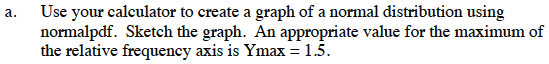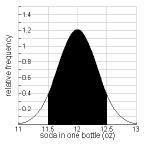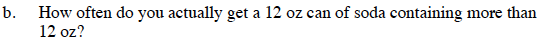The mean is the center (middle) of a normal distribution.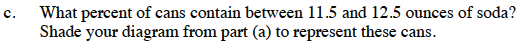normal cdf(11.5, 12.5, mean, standard deviation)